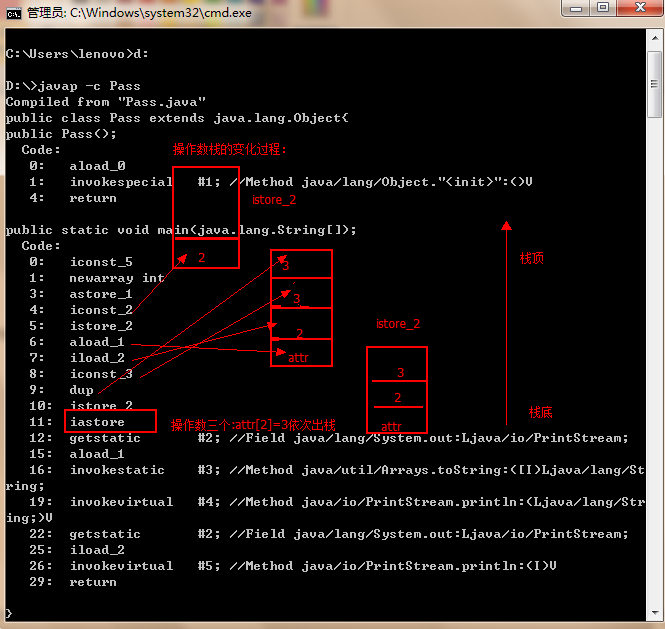Talk is cheap,show me the code.

import java.util.Arrays;
public class Test{
public static void main(String args[]){
int[] arr=new int;
int b=2;
arr[b]=b=3;
System.out.println(Arrays.toString(arr));
System.out.println(b);
}
}

[0,0,3,0,0]
3

6个回答

java运算符

1 () [] . 从左到右
2 ! +(正) -(负) ~ ++ -- 从右向左
3 * / % 从左向右
4 +(加) -(减) 从左向右
5 << >> >>> 从左向右
6 < <= > >= instanceof 从左向右
7 == != 从左向右
8 &(按位与) 从左向右
9 ^ 从左向右
10 | 从左向右
11 && 从左向右
12 || 从左向右
13 ?: 从右向左arr[b]=b=3;

int b=2;
arr[b]=b=3;